Home | | Strength of Materials II | Solved Problems: Civil - Strength of Materials - Columns

# Solved Problems: Civil - Strength of Materials - Columns

Civil - Strength of Materials - Columns

A mild steel tube 4m long, 3cm internal diameter and 4mm thick is used as a strut with  both ends hinged. Find the collapsing load, what will be the crippling load if

i.                                                                                                             Both ends are built in?

ii.                                                                                                          One end is built -in and one end is free?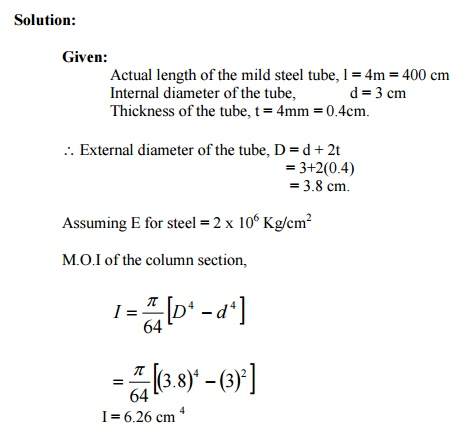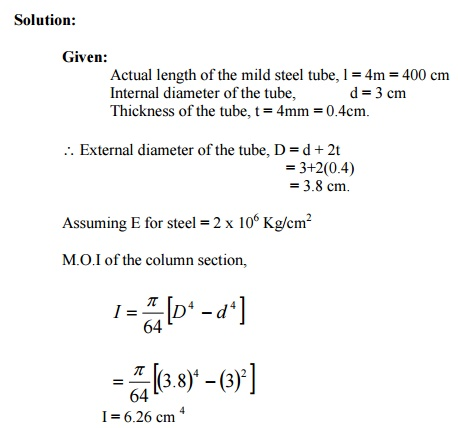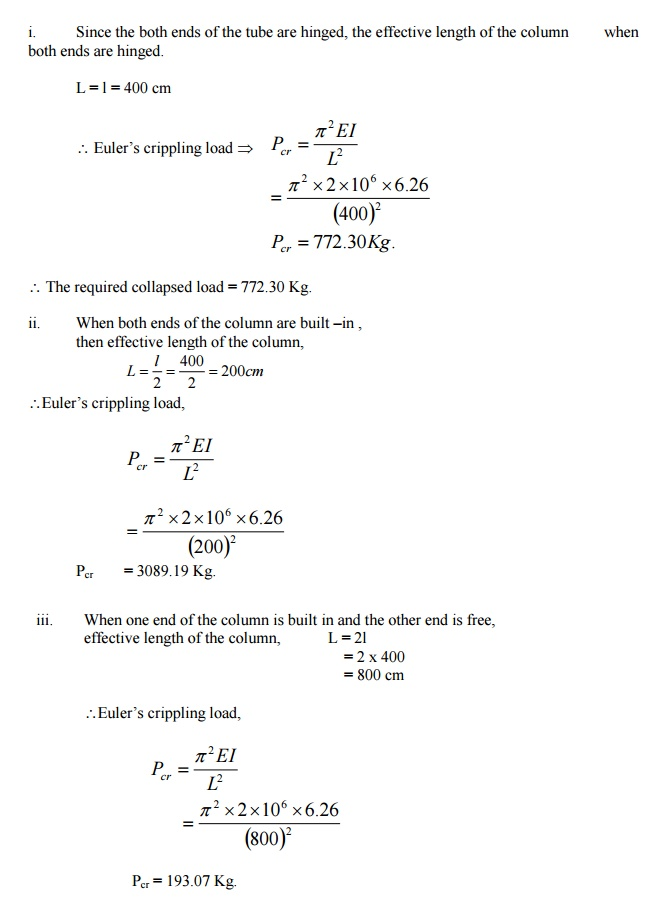A column having a T section with a flange 120 mm x 16 mm and web 150 mm x 16 mm is  3m long. Assuming the column to be hinged at both ends, find the crippling load by

6                                                                               2

using                                   Euler's   forKg/cmula..     E   =   2   x   10A steel bar of solid circular cross-section is 50 mm in diameter. The bar is pinned at both  ends and subjected to axial compression. If the limit of proportionality of the material is

210 MPa and E = 200 GPa, determine the m minimum length to which Euler'  formula is valid. Also determine the value  minimum length.A rolled steel joist ISMB 300 is to be used a column of 3 meters length with both ends fixed.  Find the safe axial load on the column. Take factor of safety 3, fc = 320 N/mm  and a = 1/7500 .  Properties of the column section.  Area = 5626 mm2,  I XX = 8.603 x 107 mm Iyy =4.539 x 107 mm4A built up column consisting of rolled steel beam ISWB 300 with two plates 200 mm x 10  mm connected at the top and bottom flanges. Calculate the safe load the column  carry, if the length is 3m and both ends are fixed. Take factor of safety 3 fc = 320 N/mm2   and a ? = 1 / 7500 Take properties of joist: A = 6133 mm2 IXX = 9821.6 x 104 mm4 ; Iyy  = 990.1 x 104  mm4A column of circular section has 150 mm dia and 3m length. Both ends of the column are  fixed. The column carries a load of 100 KN at an eccentricity of 15 mm from the  geometrical axis of the column. Find the maximum compressive stress in the  column section. Find also the maximum permissible eccentricity to avoid tension in  the column section. E = 1 x 105 N/mm2A cast iron pipe has 200 mm internal diameter and 50 mm metal thickness. It carries water  under a pressure of 5 N/mm2. Find the maximum and minimum intensities of  circumferential stress. Also sketch the distribution of circumferential stress andExplain the stresses in compound thick cylinders.

Solution:

Consider a compound thick cylinder as shown in fig.

Let,

r1=  Inner radius of the compound cylinder

r2=  Radius at the junction of the two cylinders

r3 =  Outer radius of the compound cylinder

When one cylinder is shrunk over the other, thinner cylinder is under compression and  the outer cylinder is under tension. Due to fluid pressure inside the cylinder, hoop stress will  develop. The resultant hoop stress in the compound stress is that algebraic sum of the hoop  stress due to initial shrinkage and that due to fluid pressure.

a. Stresses due to initial shrinkage: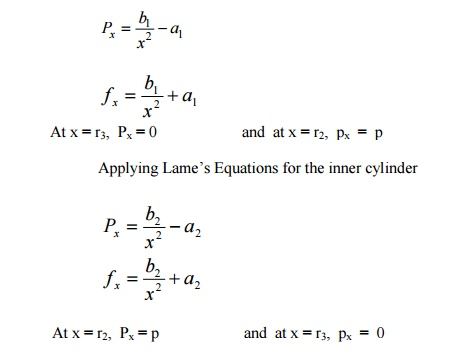b.  Stresses due to Internal fluid pressure.

To find the stress in the compound cylinder due to internal fluid pressure alone, the inner and Equation,A compound cylinder is composed of a tube of 250 mm internal diameter at 25 mm wall  thickness. It is shrunk on to a tube of 200 mm internal diameter. The radial  pressure at the junction is 8 N/mm2.

Find the variation of hoop stress across the  wall of the compound cylinder, if it is under an internal fluid pressure of 60 N/mm2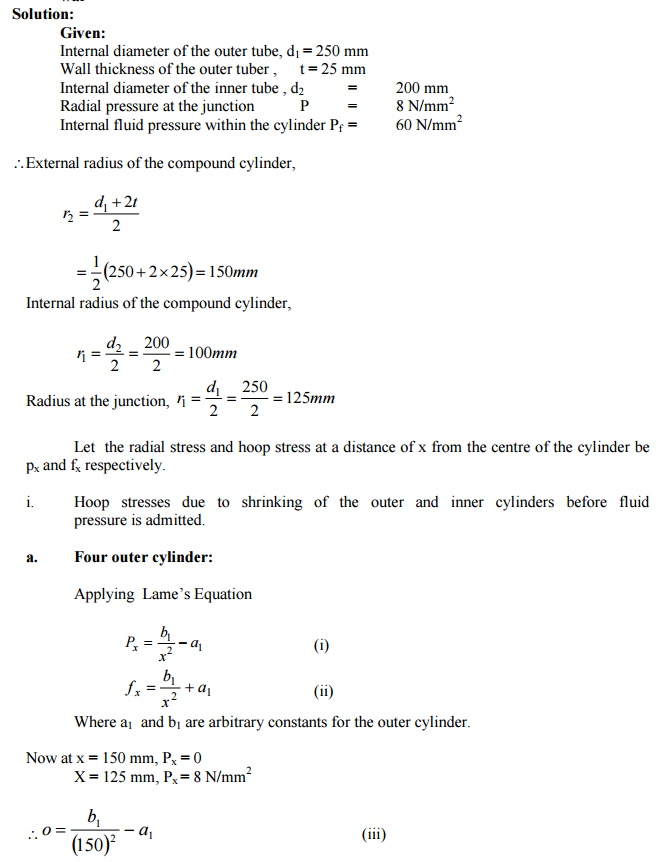Study Material, Lecturing Notes, Assignment, Reference, Wiki description explanation, brief detail
Civil : Strength of Materials : Columns : Solved Problems: Civil - Strength of Materials - Columns |